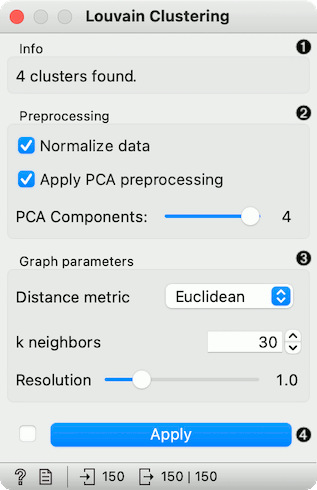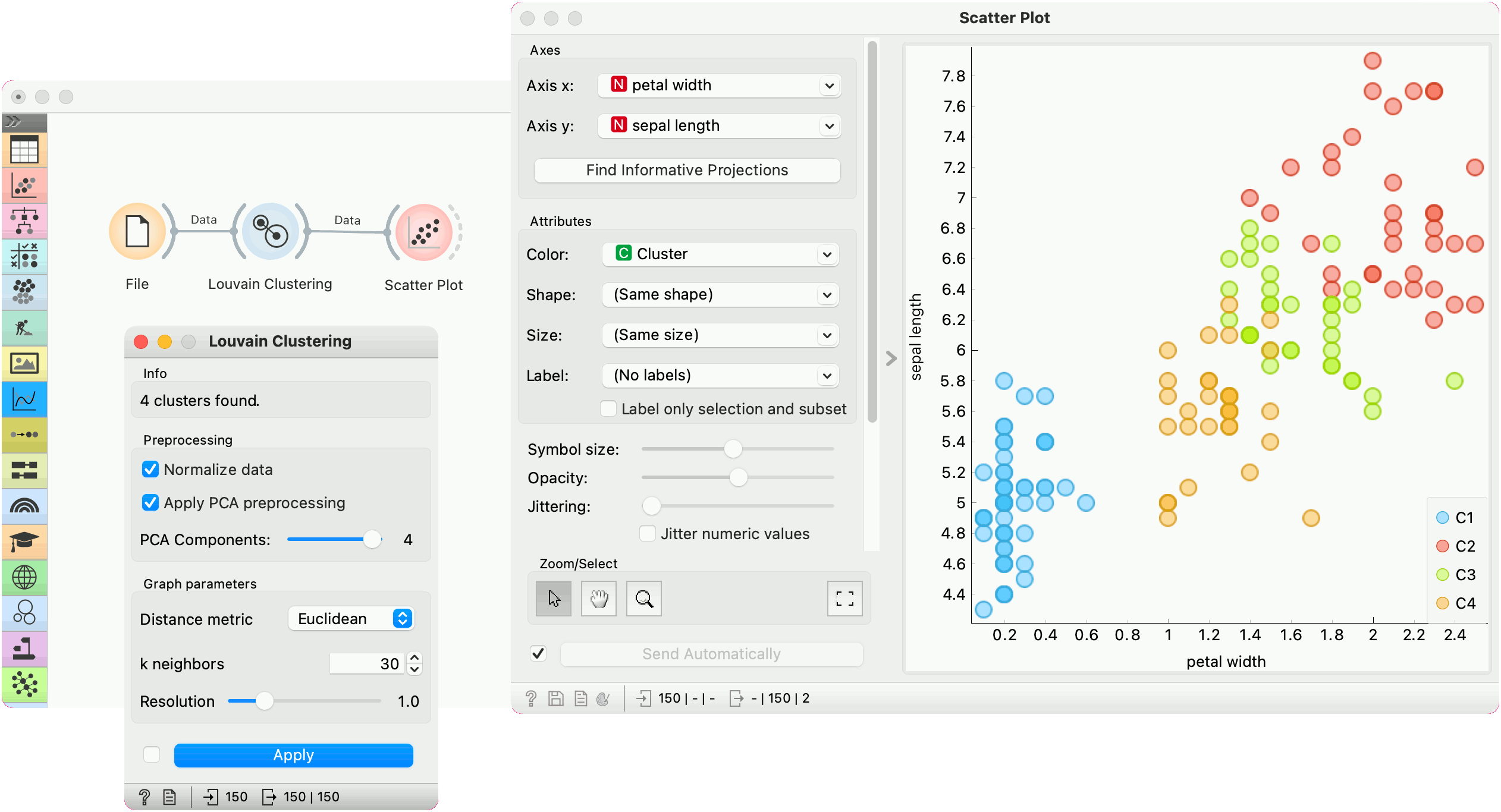# Louvain Clustering¶

Groups items using the Louvain clustering algorithm.

Inputs

• Data: input dataset

Outputs

• Data: dataset with cluster label as a meta attribute

• Graph (with the Network addon): the weighted k-nearest neighbor graph

The widget first converts the input data into a k-nearest neighbor graph. To preserve the notions of distance, the Jaccard index for the number of shared neighbors is used to weight the edges. Finally, a modularity optimization community detection algorithm is applied to the graph to retrieve clusters of highly interconnected nodes. The widget outputs a new dataset in which the cluster label is used as a meta attribute.1. Information on the number of clusters found.

2. Preprocessing:

• Normalize data: Center to mean and scale to standard deviation of 1.

• Apply PCA preprocessing: PCA processing is typically applied to the original data to remove noise (see PCA widget).

• PCA Components: number of principal components used.

3. Graph parameters:

• Distance metric: The distance metric is used for finding specified number of nearest neighbors (Euclidean, Manhattan, Cosine).

• k neighbors: The number of nearest neighbors to use to form the KNN graph.

• Resolution is a parameter for the Louvain community detection algorithm that affects the size of the recovered clusters. Smaller resolutions recover smaller clusters and therefore a larger number of them, while, conversely, larger values recover clusters containing more data points.

4. When Apply Automatically is ticked, the widget will automatically communicate all changes. Alternatively, click Apply.

## Preprocessing¶

Louvain Clustering uses default preprocessing if necessary. It executes it in the following order:

• continuizes categorical variables (with one feature per value)

• imputes missing values with mean values

To override default preprocessing, preprocess the data beforehand with Preprocess widget.

## Example¶

Louvain Clustering converts the dataset into a graph, where it finds highly interconnected nodes. In the example below, we used the iris data set from the File widget, then passed it to Louvain Clustering, which found 4 clusters. We plotted the data with Scatter Plot, where we colored the data points according to clusters labels.We can visualize the graph itself using the Network Explorer from the Network addon.